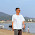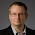## 2015. május 26., kedd

### Introduction

In this final part about subscription-containers, I'm going to demonstrate an array-backed container based on the copy-on-write approach and atomics.

Why is this type of container so important? My answer is another question: if the contained subscriptions were Subscribers, what would you do with an array of Subscribers?

You could implement operators requiring multicast to child subscribers with it and maintain the thread-safety and termination guarantees, similar to how RxJava's Subjects handle their subscribers and more importantly, how the - recently rewritten - publish() does it too.

### Array-backed container

Let's build such a container with the following requirements: ability to add and remove some kind of subscriptions (i.e., Subscribers), ability to get the current contents, ability to 'unsubscribe' the container without unsubscribing the contents and the ability to tell if an add succeeded or not.

The class skeleton, with the trivial implementations already filled in, looks like this:

```@SuppressWarnings({"rawtypes", "unchecked"})                 // (1)
public class SubscriberContainer<T> {
static final Subscriber[] EMPTY = new Subscriber;     // (2)
static final Subscriber[] TERMINATE = new Subscriber;

final AtomicReference<Subscriber[]> array
= new AtomicReference<>(EMPTY);                      // (3)

public Subscriber<T>[] get() {                           // (4)
return array.get();
}

public boolean add(Subscriber<T> s) {                    // (5)
// implement
}

public boolean remove(Subscriber<T> s) {                 // (6)
// implement
}

public Subscriber<T>[] getAndTerminate() {               // (7)
return array.getAndSet(TERMINATE);
}

public boolean isTerminated() {                          // (8)
return get() == TERMINATED;
}
}```

The structure consists of elements as follows:

1. Java doesn't allow generic base types for arrays and doesn't like certain type conversions so we are forced to suppress the warnings regarding raw types and converting those raw values back to parametric types. Generally, the implementation has to be safe but the user of the class will remain typesafe.
2. We use a constant array for indicating the empty state and the terminated state.
3. We store the current array in an AtomicReference instance, but if you know the class won't be extended further, you can just extend AtomicReference directly.
4. The get() method returns the current array of contained items. For performance reasons, one should use this array in read-only manner (otherwise, defensive copies are required on each invocation).
5. The add() method takes a properly-typed Subscriber and returns true if it could be added or false if the container was terminated.
6. The remove() method tries to remove the given subscriber instance and returns true if successful.
7. Instead of a plain unsubscribe() method, we do a handy trick: the current array is replaced with the terminal indicator and return the previous array. It becomes useful when an atomic termination and post-termination actions are needed.
8. Since a regular empty array can't be told apart from the terminated state, a method is needed which checks for this terminal state explicitly.
The add() method will be the simpler one:

```    // ...
public boolean add(Subscriber<T> s) {
for (;;) {
Subscriber[] current = array.get();
if (current == TERMINATED) {                  // (1)
return false;
}
int n = current.length;
Subscriber[] next = new Subscriber[n + 1];
System.arraycopy(current, 0, next, 0, n);     // (2)
next[n] = s;
if (array.compareAndSet(current, next)) {     // (3)
return true;
}
}
}
// ...
```

Here we have a classical CAS loop:

1. If the container contains the terminated token, the method returns with false and the subscriber is not added to the container. The caller can then decide what to do with said subscriber (i.e., send onCompleted event to it).
2. A copy of the current contents are made into an array with length + 1 and the subscriber is assigned to the very end.
3. The CAS will try to 'commit' the changes and the method returns true. Otherwise, a new attempt is made.

Lastly, the remove() looks like as follows:

```    // ...
public boolean remove(Subscriber<T> s) {
for (;;) {
Subscriber[] current = array.get();
if (current == EMPTY
|| current == TERMINATED) {             // (1)
return false;
}
int n = current.length;
int j = -1;
for (int i = 0; i < n; i++) {                   // (2)
Subscriber e = current[i];
if (e.equals(s)) {
j = i;
break;
}
i++;
}
if (j < 0) {                                    // (3)
return false;
}
Subscriber[] next;
if (n == 1) {                                   // (4)
next = EMPTY;
} else {
next = new Subscriber[n - 1];
System.arraycopy(current, 0, next, 0, j);
System.arraycopy(current, j + 1,
next, j, n - j - 1);                    // (5)
}
if (array.compareAndSet(current, next)) {       // (6)
return true;
}
}
}

```
Although a bit complicated, the method's behavior is straightforward:

1. In case the current array is empty or the container is already terminated, it can't contain any subscribers and thus the method quits immediately.
2. Otherwise, we search for the first occurrence of the given subscriber and hold onto its index in j. By scanning first instead of doing an on-the-fly filter-copy, we can save some overhead due to the card-marking associated with each reference store, required by most GCs.
3. If j remained negative, the subscriber is not amongst the others in the array and the method returns false.
4. In case the array contains a single value, there is no need to create an empty array but we can reuse the constant (since empty arrays are essentially stateless).
5. If the array contains multiple elements, a new shorter array is created and values around the found location is then copied over to it.
6. Finally, the CAS will swap in the new array and returns true indicating the subscriber was successfully removed.

Such a container is quite often used in multicast-like operators but these operators rarely encounter more than half-dozen subscribers during their lifecycle and as such, the frequent allocation of arrays has less impact.

If the allocation rate is a problem in your scenario, it is possible to change the above logic to use synchronized block and some type of list or set to store the subscriptions, but note that the get() method, quite frequently used when dispatching events, can no longer be implemented in a wait-free manner. The get() method will, most likely, require the use of a synchronized block and making defensive copies for every invocation; again, a tradeoff one needs to consider carefully.

### Conclusion

In this mini-series, I talked about various kinds of standard subscription containers and shown how to implement blocking and lock-free containers. In addition, I've described an array-backed lock-free container with peculiar properties that come in handy when implementing multicast-like operators.

If there was only RxJava 1.x, the operator concurrency primitives series could end here. However, the reactive-streams has been recently finalized and is expected to form the basis for RxJava 2.0 and that is going to be a complete rewrite; it is unavoidable.

Does this mean the things learned so far are useless? Yes and no. The concepts will be quite relevant in fact, only the class structures need to change somewhat to match the reactive-streams specification and requirements.

Instead of jumping right into RxJava 2.0 constructs, let's take a break and look at some other advanced RxJava topics: schedulers

#### 2 megjegyzés:

1.In the explanation part of `remove()` method, point 6: "Finally, the CAS will swap in the new array and returns true indicating the subscriber was successfully added.", it should indicate "the subscriber was successfully removed."

1.Thanks. Fixed.